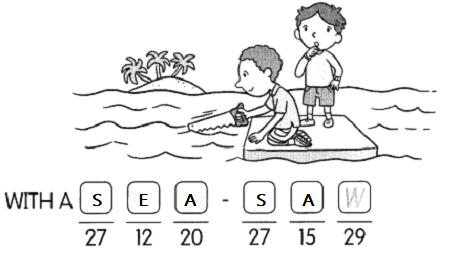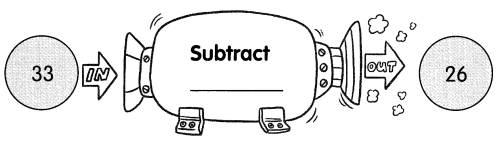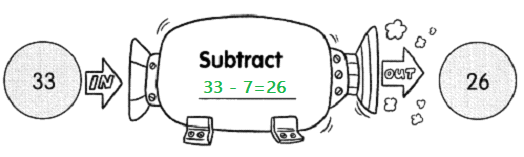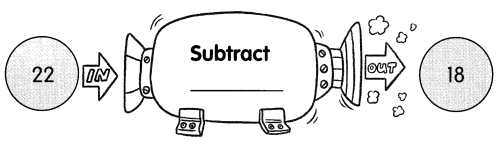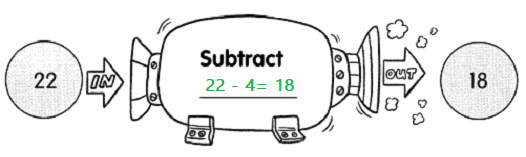# Math in Focus Grade 1 Chapter 13 Practice 4 Answer Key Subtraction with Regrouping

Practice the problems of Math in Focus Grade 1 Workbook Answer Key Chapter 13 Practice 4 Subtraction with Regrouping to score better marks in the exam.

## Math in Focus Grade 1 Chapter 13 Practice 4 Answer Key Subtraction with Regrouping

Regroup.

Question 1.
27 = 1 ten ________ ones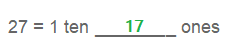Question 2.
15 = 0 tens ________ ones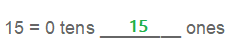Question 3.
30 = 2 tens ________ ones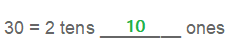Subtract.

Question 4.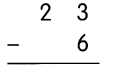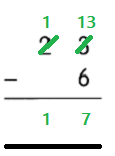Question 5.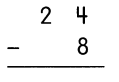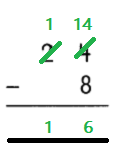Question 6.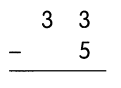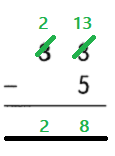Question 7.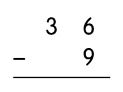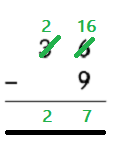Question 8.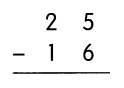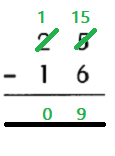Question 9.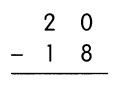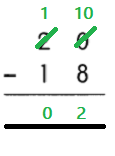Fill in the missing numbers.

Question 10.
21 – 5 = ___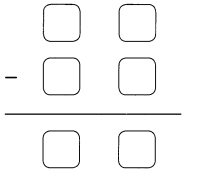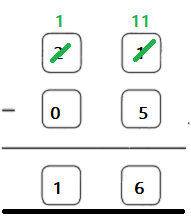Question 11.
36 – 7 = ___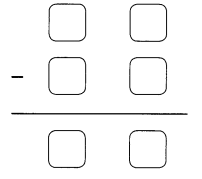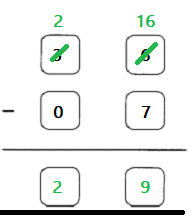Question 12.
25 – 18 = ___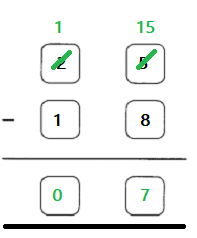Question 13.
31 – 18 = ___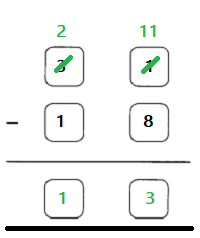Question 14.
32 – 14 = ___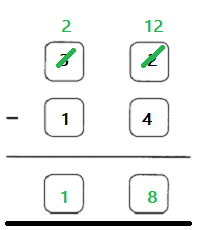Question 15.
30 – 17 = ___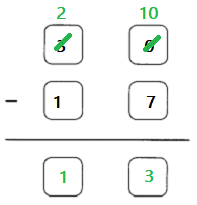Subtract.

Then solve the riddle.

Question 16.
38 – 9 = 29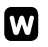Question 17.
30 – 18 = _____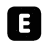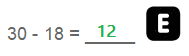Question 18.
32 – 5 = ___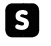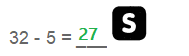Question 19.
35 – 8 = ___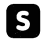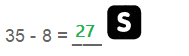Question 20.
27 – 7 = _____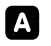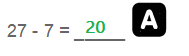Question 21.
34 – 19 = _____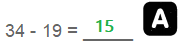How do you cut the sea?

Match the letters to the answers below to find out.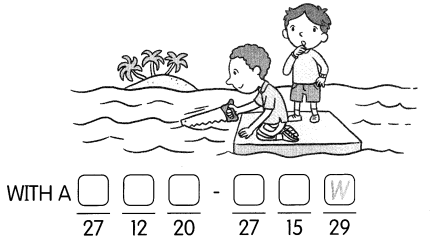Nafasha drops a ball into each number machine. Write the missing numbers in the blanks to show what happens to each ball.